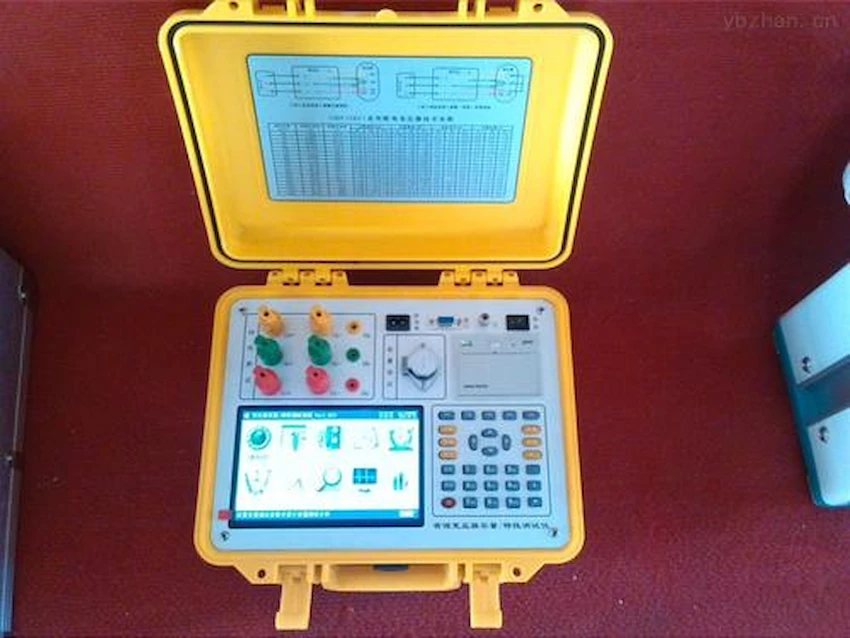We can provide you single phase and three phase pad mounted transformer

#### Dry-type Transformer

Type：Cast resin; Rated Capacity: Up to 25MVA; Rated Voltage: Up to 36KV;

#### Pole Transformer

TypeCSP type Frequency: 50/60Hz; Rated Power: 5~167kva

#### Dry-type Transformer

Frequency: 50/60Hz Rated voltage:10kv, 20kv,30kv Rated Power: 400~2500kva

# How to calculate the power losses of the transformer, the transformer losses formula iron losses and copper losses power

## Calculation Method of Power Transformer Losses

Transformer losses refers to the sum of no-load loss Po, short-circuit loss Pk and stray loss Ps. The no-load loss and load loss of the transformer refer to iron losses and copper losses respectively.

What is the no load losses of transformer？The power losses of the transformer is composed of iron loss and copper loss. The iron loss is related to the running time, and the copper loss is related to the load.

Then, when calculating the power losses of the transformer, the iron losses and the copper losses need to be calculated separately.

### 1. Calculation of iron losses power

Iron loss power of different models and capacities, calculation transformer losses formula

: iron loss power (kWh) = no-load loss (kW) × power supply time (hours)

The no-load loss (iron loss) of the distribution transformer can be found in the attached table. The power supply time is the actual operating time of the transformer, which is determined according to the following principles:

(1) For users with continuous power supply, the whole month is calculated as 720 hours.

(2) Intermittent power supply or curtailment due to power grids shall be calculated according to the actual power supply hours of the substation to the user. The calculation shall not be based on the difficulty of calculation. The calculation shall be based on the operation of the whole month. Time should be deducted when calculating the iron loss.

(3) Users who have a product clock on the low-voltage side of the transformer are calculated according to the accumulated power supply time of the product clock.

## 2. Calculation of copper losser power

When the load factor is 40% and below, it will be charged as 2% of the monthly electricity consumption (using the meter reading). The calculation transformer losses formula: copper loss electricity (kWh) = monthly electricity consumption (kWh) × 2%

Copper loss is related to the size of the load current (electricity). When the monthly average load rate of the distribution transformer exceeds 40%, the copper loss electricity should be calculated at 3% of the monthly electricity consumption. The monthly electricity consumption when the load factor is 40% is checked by the attached table.

The calculation transformer losses formula of load rate: load rate = copy see electricity/S.T.Cos￠

In the formula: S——Rated capacity of distribution transformer (kVA); T——Full month calendar time, 720 hours;

COS￠——power factor, take 0.80.

The transformation loss of a power transformer is divided into copper loss and iron loss. Copper loss is generally 0.5%. The iron loss is generally 5~7%. The transformation loss of dry-type transformers is smaller than that of oil-invaded transformers. Total variable loss: 0.5+6=6.5

Calculation method: 1000KVA×6.5%=65KVA, 65KVA×24 hours×365 days=569400KWT (degrees)

The specific data will be marked on the nameplate on the transformer, so you can take a look at it when you have time.

The above is the calculation method of the power losses of the transformer, I hope it will be helpful to everyone.

### 5 Responses

1.Hairstyles says:

Together with everything which appears to be building throughout this specific subject matter, your points of view are actually quite refreshing. However, I beg your pardon, but I do not give credence to your whole strategy, all be it exhilarating none the less. It appears to everybody that your opinions are actually not totally validated and in reality you are yourself not even thoroughly certain of the assertion. In any case I did appreciate looking at it.

2.SUPERSLOT says:

Fantastic post.Really looking forward to read more. Cool.

3.Hairstyles says:

I appreciate, cause I found just what I was looking for. You have ended my 4 day long hunt! God Bless you man. Have a great day. Bye

4.Latest Hairstyles says:

Valuable information. Lucky me I found your web site by accident, and I am shocked why this accident did not happened earlier! I bookmarked it.

5.Heriberto Krall says:

What a data of un-ambiguity and preserveness of precious knowledge regarding unpredicted emotions.

## Share:

### 10 kv Oil Filled Transformer

Fault Diagnosis and Analysis of 10 kv Oil Filled Transformer 10 kv Oil filled transformer is a key equipment for power distribution, and its failure